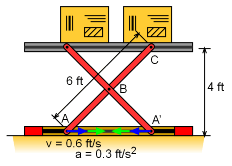Ch 5. Rigid Body General Motion Multimedia Engineering Dynamics Fixed Axis Rotation Plane Motion Velocities Zero Velocity Point Plane Motion Accelerations Multiple Gears Rot. Coord. Velocities Rot. Coord. Acceleration
 Chapter - Particle - 1. General Motion 2. Force & Accel. 3. Energy 4. Momentum - Rigid Body - 5. General Motion 6. Force & Accel. 7. Energy 8. Momentum 9. 3-D Motion 10. Vibrations Appendix Basic Math Units Basic Equations Sections Search eBooks Dynamics Fluids Math Mechanics Statics Thermodynamics Author(s): Kurt Gramoll ©Kurt GramollDYNAMICS - CASE STUDY IntroductionProblem Diagram A new dock lift is being used to move delicate computer equipment off shipping trucks. You need to know what the acceleration of the boxes is when the boxes are at dock level. What is known: The bottom loading pistons accelerate horizontally at a constant 0.3 ft/s2. The horizontal velocity of the pistons is 0.6 ft/s when the lift is 4 ft high. The lifting arms (AC) is 6 ft long. Point A and A' are constrained to move horizontally. Point B is constrained to move vertically. Question What is the vertical acceleration of the lift's platform when it reaches the top of the dock? Approach Use the relative velocity equation vB = vA + vB/A (see piston problem) to determine the angular velocity of the lifting bar AC. This is needed to solve for the accelerations. Use the relative acceleration equation to find the acceleration of point A. aB = aA + aB/A

Practice Homework and Test problems now available in the 'Eng Dynamics' mobile app
Includes over 400 problems with complete detailed solutions.
Available now at the Google Play Store and Apple App Store.